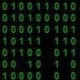# 打印质数的各种算法

• 实际应用和教学应用有很大的差别。
• 最后的那个使用编译时而不是运行时的方法大家可以重点看看。

#### 教科书的示例

```#include <iostream>
using namespace std;

bool isPrime(int nr)
{
for (int d = 2; (d * d) < (nr + 1); ++d){
if (!(nr % d)){
return false;
}
}
return true;
}

int main (int argc, char * const argv[])
{
for (int i = 0; i < 50; ++i){
if (isPrime(i)){
cout << i << endl;
}
}
}
```

#### 较好的算法

` 2  3  4  5  6  7  8  9 10 11 12 13 14 15 16 17 18 19 20 21 22 23 24 25 26 27 28 29 30`

` 2  3     5     7     9    11    13    15    17    19    21    23    25    27    29`

` 2  3     5     7          11    13          17    19          23    25          29`

` 2  3     5     7          11    13          17    19          23                29`

#### 使用编译时而不是运行时

```template<int N, int D = N - 1>
struct isPrime {
enum {
result = (N % D) && isPrime<N, D-1>::result
};
};

template<int N>
struct isPrime<N, 1> {
enum {
result = true
};
};
```

```if (isPrime<3>::result)
cout << "Guess what: 3 is a prime!";
```

```template<int N, bool ISPRIME>
struct printIfPrime {
static inline void print() {}
};

template <int N>
struct printIfPrime<N, true> {
static inline void print() {
std::cout << N << endl;
}
};
```

```template<int N, int MAX>
struct printPrimes {
static inline void print()
{
printIfPrime<N, isPrime<N>::result>::print();
printPrimes<N + 1, MAX>::print();
}
};
```

```template<int N>
struct printPrimes<N, N> {
static inline void print() {
printIfPrime<N, isPrime<N>::result>::print();
}
};
```

```int main (int argc, char * const argv[])
{
printPrimes<2, 40>::print();
return 0;
}
```

• 比较耗编译时间。
• 不能在运行时输入MAX的值。

（全文完）（转载本站文章请注明作者和出处 酷 壳 – CoolShell ，请勿用于任何商业用途）

——=== 访问 酷壳404页面 寻找遗失儿童。 ===——(10 人打了分，平均分： 4.00 )Loading...

## 《打印质数的各种算法》的相关评论

1.Nemo说道：

还是经典的筛选法高效啊

2.tinybit说道：

很好的文章，特别是第二个算法，很实际。第三个很Geeker。

另外，教科书实例中循环 for (int d = 2; (d * d) <= (nr + 1); ++d)
似乎循环到 (d * d) <= nr; 就可以结束了，为什麽要到nr + 1呢？

3.WindyWinter说道：

@tinybit
nr=3

4.WindyWinter说道：

存文件和运行时计算是要权衡的，有时候数据大到蛋疼，但生成算法很简单，那即便生成比较费时，也只能认了。

5.tinybit说道：

@WindyWinter
nr=3的时候，也没什么问题啊。
如果nr不是质数，必然有两个大于1的正整数（质因数），使得p*q=nr, 进一步得出p和q中必须有一个位于区间[2, 根号(nr)]上，因此只需循环到 (d * d) <= nr 就可以断定是否存在这样的质因数。

6.digiter说道：

第一个算法是O(sqrt(n))的吧，怎么会接近O(nlog(n))呢？如果是O(nlog(n))的，那RSA就不安全了

7.digiter说道：

@digiter
给个O(n)筛素数的方法：

```const int size = 1000 * 1000 + 5;
bool isp[size];
int prime[size], lp;
void make() {
memset(isp, true, sizeof(isp));
isp = isp = false;
lp = 0;
for (int i = 2; i < size; ++i) {
if (isp[i]) {
prime[lp++] = i;
}
for (int j = 0; j < lp && (long long)i * prime[j] < size; ++j) {
isp[i * prime[j]] = false;
if (i % prime[j] == 0) {
break;
}
}
}
}
```
8.夜弓说道：

cpp template的方法火星了

9.bigeast说道：

tinybit :
很好的文章，特别是第二个算法，很实际。第三个很Geeker。
另外，教科书实例中循环 for (int d = 2; (d * d) <= (nr + 1); ++d)
似乎循环到 (d * d) <= nr; 就可以结束了，为什麽要到nr + 1呢？

有的版本是先算出sqrt(n),但是这样会有精度损失，为了以防万一会故意另i<sqrt(i+1.0)时循环终止，我想这个+1的风格可能是这样延续下来的。。

10.bigarm说道：

较好的算法，那一节第二行，有个错误：写成”级数级的算法”了，应该是对数级的算法log(n)

1.陈皓说道：

谢谢指正。

11.Astrum说道：

所以，我想在这里提醒大家——实际和理论的的方法很不一样的，千万不要读书读成书呆子。在游戏编程的世界里，大量的数据都不是运行计算的，而都是写在文件中的。比如，一个火焰效果，一个人物跑动的动作，都是事先写在文件中的。
————————
这一段有点小问题，为了追求真实度，现在的游戏特效也大部分是用gpu实时演算的，像光影，爆炸，碰撞，等等。 纯挑刺纯挑刺~哈哈~

1.陈皓说道：

欢迎挑刺！谢谢啊，这让我也对游戏开发有了很多的了解！

12.j说道：

充电…

13.Michael Genn说道：

C++ Template Metaprogramming!!!

14.funny说道：

第一种方法求单个是否是素数时间复杂度是O(sqrt(n))而0-n是O(n*sqrt(n) 第二种均摊o(n)但是其直接求出0-N所有的素数 但是其最大的问题是需要开辟额外的内存 面对大素数鸭梨很大 另外有2种介于第一种和第二种直接的求素数的方式费马测试 和 米勒测试 比较犀利

15.jerry说道：

夜弓 :
cpp template的方法火星了

顶。现在最骨灰级的用法都在boost和0x里面了。

16.T.D.M.说道：

受益匪浅！

17.N/A说道：

@tinybit
根据质数的定义，在判断一个数N是否是质数时，我们只要用1至N – 1去除N，看看能否整除即可。但我们有更好的办法。先找一个数M，使M的平方大于N，再用 <= M的质数去除N（N即为被除数），如果都不能整除，则N必然是质数。

18.JinCeon说道：

tinybit :
很好的文章，特别是第二个算法，很实际。第三个很Geeker。
另外，教科书实例中循环 for (int d = 2; (d * d) <= (nr + 1); ++d)
似乎循环到 (d * d) <= nr; 就可以结束了，为什麽要到nr + 1呢？

@tinybit <nr+1和<=nr不是一样吗

19.walfud说道：

虽然 1 既不是质数也不是合数, 但是我还是写错了, 写了printPrime 会由于
isPrime 而导致编译死循环( -1 被解释为 unsigned int, 由于各编译器嵌套深度上线不一样, 所以所得的值也不一样), 我尝试在 enum 中加入 ?: 表达式, 未果, 修改方法如下:
template
struct isPrime
{
enum {result = 2 < N ?
N % D && isPrime::result : false} ;
} ;
请问楼主有什么好方法能防止上述情况?

20.dent说道：

这里讨论的是最基本的trial division法，更大的质数用这种方法效率就很低了，看了下wiki上质数的段落，检测大质数的算法真麻烦，需要有很好的数学功底。

21.水长东说道：

刷选法肯定快些。

22.怪兽说道：

我们发现在2，3，5，7这4个数了没有如果一个数是素数肯定是2，3，5，7这几个数组成的。

23.ethantsien说道：

第一个算法的复杂度应该是logN，而不是N*logN

24.hjj说道：

“较好的算法”实际上还有提高空间，可以用一个素数列表保存已筛选出的素数，对后面的每个数n，使用 <= sqrt(n) 的素数筛选，这样算法复杂度不变，空间占用从 O(N) 降到了 O(N/lnN)。

25.wxd356说道：

digiter :
@digiter
给个O(n)筛素数的方法：
12345678910111213141516171819`const` `int` `size = 1000 * 1000 + 5;``bool` `isp[size];``int` `prime[size], lp;``void` `make() {``    ``memset``(isp, ``true``, ``sizeof``(isp));``    ``isp = isp = ``false``;``    ``lp = 0;``    ``for` `(``int` `i = 2; i < size; ++i) {``        ``if` `(isp[i]) {``            ``prime[lp++] = i;``        ``}``        ``for` `(``int` `j = 0; j < lp && (``long` `long``)i * prime[j] < size; ++j) {``            ``isp[i * prime[j]] = ``false``;``            ``if` `(i % prime[j] == 0) {``                ``break``;``            ``}``        ``}   ``    ``}``}`

镶嵌for难道不是O(n^2)吗？反正我想不出来怎么可能是O(n)

26.受到了风说道：

我觉得isPrime中的result按照惯用应该改成value，最后的那个模板特化中的N改成MAX应该更好理解，毕竟编译的递归是在N+1=MAX时停止的。

27.受到了风说道：

而且isPrime没有处理N=1的情况，如果N=1，那么D=0，所以应该再加个模板特化
template
struct is_prime
{
enum{value = false};
};
template
struct is_prime
{
enum{value = false};
};

同理，对于N为负数的情况，也没有做太多处理，所以isPrime的N的类型应该改为size_t

28.unknown说道：

@wxd356
注意那个 if (i % prime[j] == 0) 所以每个数只被他最小的质因子筛掉一次

29.listar说道：

那个模板的方法，只能在编译时求解有限个数的质数，因为编译器对模板的递归调用是有限制的，比如clang++的，fatal error: recursive template instantiation exceeded maximum depth of 256